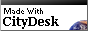Home

 hello CUDA: a grid of blocks x threads - GPU series #4 Tuesday,  01/24/23  08:25 PMAnother day, another post about CUDA and GPU acceleration.  Now we're going to build on the detailed example from yesterday, in which we multi-threaded a simple example.  We'll extend this to run a parallel grid with multiple blocks of multiple threads.  (Series starts here, next post here)

Previously we saw that we could easily run many threads in parallel:Up to 1,024!  But what if we want to run even more?

Turns out GPUs enable many blocks of threads to be run in parallel, like this:Many (many!) blocks of threads can be invoked, and the GPU will run as many of them as possible in parallel.  (The exact number which will run depends on the GPU hardware.)

Let's see what this looks like in code, here is hello4.cu:As before the changes are highlighted.  We've added a new parameter gpublocks to specify the number of blocks.  If this is given as zero, we compute the blocks as arraysize / gputhreads.

We've specified gpublocks as the first parameter in the triple-angle brackets, on the kernel invocation of domath().  Remember that the second parameter is the number of theads per block, so the total parallel threads is block x threads.

And we've changed the way the index and stride are computed inside the domath() function, so that the array is parcelled out to all the threads in all the blocks.  You'll note this makes use of several global variables provided by CUDA: threadId, blockDim, and now also blockId and gridDim.

So what will happen now?  Let's try running hello4:Wow.  With 10 blocks of 1,024 threads (10,240 threads overall), the runtime goes down to 2.3s.  And if we compute the maximum number of blocks (by specifying 0 as the parameter), we get 12,056 blocks of 1,024 threads, for a runtime of .4s!  That's GPU parallelism in action, right there.

Furthermore, when we specify an additional order of magnitude to make the array size 123,456,789, we run 120,563 blocks of 1,024 threads, and the total runtime of that is 3.7s.  Way way better than CPU only (hello1) which was 50s!

In fact, something interesting about this run, the array allocation took most of the time; the actual computation only required .16s.  Which is a good segue to the next discussion, about memory, and we'll tackle that in the next installment.